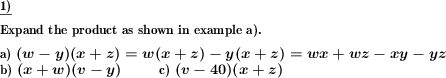Custom math worksheets at your fingertips# Details for problem "Product of two sums term expansion"

Quickname: 1595

Junior High School, Middle School, High School.

## Summary

Expand a product term which has sums with variables as factors.

## Example## Description

A product term whose factors are a product or number and a sum has to be expanded. The term may contain up to three variables.

The shape of the term to be expanded may be one of these:

a) (a+b)(c+d)

b) (a+b)(c-d)

c) (a-b)(c+d)

d) (a-b)(c-d)

The term can be chosen to be

• one of group a, which means both factors are sum terms.
• one of groups a,b or c, which means at most one factor is a difference term.
• any one of groups a-d, which means both factors can be difference terms
• one of groups b,c or d, which means at least one factor is a difference term.

Further,

• squares can be disallowed, which means a variable will appear only in one of the factor terms.
• it can be specified that one of the variables in each factor may be a number.

The range for the numbers can be chosen.

The number of problems is selectable. The first problem can be presented with the answer in the form of a sample problem. If required, some space will be left so that the answer may be written on the sheet.

Download free printable worksheets for this math problem here. The worksheet contains the problems only, the solution sheet includes the answers. Just click on the respective link.

•Worksheet 1Solution sheet with answers
•Worksheet 2Solution sheet with answers
•Worksheet 3Solution sheet with answers

If you can not see the solution sheets for download, they may be filtered out by an ad blocker that you may have installed. If this is the case, please allow ads for this page and reload the page. The solution sheets will then reappear.

• Do these sample worksheets do not really fit?
• Do you need more math worksheets, with a different level of difficulty?
• Would you like to combine different problems on a worksheet and adjust them to your needs?
• As a teacher, you can put together your own worksheets using the automatically generated math problems provided.
With a free initial credit, you can start creating your own math worksheets in a few minutes.

You can try it for free! Register here, to create custom worksheets now!

## Customization options for this problem

Parameter
Possible values
Number of problems
1, 2, 3, 4, 5, 6, 7, 8, 9, 10
Number Range
20, 50, 99, 200, 499, 999, 1999, 4999
Problem type
(a+b)(c+d), (a-b) allowed, exactly one of (a-b), at least one (a-b)
Sample problem
Yes, No
Yes, No
Allow squares
Yes, No
Numbers allowed
Yes, No

## Similar problems

Remark
Description
Expand product of product and sum term
Expand product term with variables.